# Sphere Problems

#### Number of problems found: 112

• Hollow sphereCalculate the weight of a hollow stříbrné sphere (density 10.5 g/cm3) if the vnitřní diameter is 15 cm and wall thickness is 1 mm.
• Iron sphereIron sphere has weight 100 kg and density ρ = 7600 kg/m3. Calculate the volume, surface and diameter of the sphere.
• Sphere equationObtain the equation of sphere its centre on the line 3x+2z=0=4x-5y and passes through the points (0,-2,-4) and (2,-1,1).
• Inscribed sphereHow many % of the volume of the cube whose edge is 6 meters long is a volume of a sphere inscribed in that cube?
• Spheres in sphere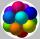How many spheres with a radius of 15 cm can fit into the larger sphere with a radius of 150 cm?The surface of the sphere is 60 cm square. Calculate its radius; result round to tenth of cm.
• Sphere and coneWithin the sphere of radius G = 33 cm inscribe the cone with the largest volume. What is that volume, and what are the dimensions of the cone?
• Sphere in coneA sphere of radius 3 cm describes a cone with minimum volume. Determine cone dimensions.
• Half-sphere roofThe roof above the castle tower has the shape of a 12.8 m diameter half-sphere. What is the cost of this roof, if the cost of 1 square meter is 12 euros and 40 cents?
• SphereThe surface of the sphere is 12100 cm2, and the weight is 136 kg. What is its density?
• Cube from sphere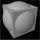What largest surface area (in cm2) can have a cube that was cut out of a sphere with radius 43 cm?
• Equilateral cylinder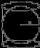A sphere is inserted into the rotating equilateral cylinder (touching the bases and the shell). Prove that the cylinder has both a volume and a surface half larger than an inscribed sphere.
• Spherical capFrom the sphere of radius 11 was truncated spherical cap. Its height is 6. What part of the volume is a spherical cap from the whole sphere?
• A spherical segment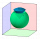A spherical section whose axial section has an angle of j = 120° in the center of the sphere is part of a sphere with a radius r = 10 cm. Calculate the cut surface.
• Tangent spheresA sphere with a radius of 1 m is placed in the corner of the room. What is the largest sphere size that fits into the corner behind it? Additional info: Two spheres are placed in a corner of a room. The spheres are each tangent to the walls and floor and
• Sphere slicesCalculate the volume and surface of a sphere if the radii of parallel cut r1=31 cm, r2=92 cm, and its distance v=25 cm.
• How many 9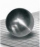How many percent volume remain of a sphere if diameter decrease 3×?
• Spherical capPlace a part of the sphere on a 4.6 cm cylinder so that the surface of this section is 20 cm2. Determine the radius r of the sphere from which the spherical cap was cut.
• Sphere parts, segmentA sphere with a diameter of 20.6 cm, the cut is a circle with a diameter of 16.2 cm. .What are the volume of the segment and the surface of the segment?
• Cube in a sphereThe cube is inscribed in a sphere with a volume 7253 cm3. Determine the length of the edges of a cube.

Do you have an interesting mathematical word problem that you can't solve it? Submit a math problem, and we can try to solve it.

We will send a solution to your e-mail address. Solved examples are also published here. Please enter the e-mail correctly and check whether you don't have a full mailbox.

Please do not submit problems from current active competitions such as Mathematical Olympiad, correspondence seminars etc...